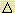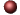Chemical EquilibriumExamples ofMultiple Choice Questions

1.
When the system A + BC + D is at equilibrium,
(a) the sum of the concentrations of A and B must equal the sum of the concentrations of C and D.
(b) the forward reaction has stopped.
(c) both the forward and the reverse reactions have stopped.
(d) the reverse reaction has stopped.
(e) neither the forward nor the reverse reaction has stopped.

2.
2SO3(g)2SO2(g) + O2(g)
The conventional equilibrium constant expression (Kc) for the system as described by the above equation is:
(a) [SO2]2/[SO3]
(b) [SO2]2[O2]/[SO3]2
(c) [SO3]2/[SO3]2[O2]
(d) [SO2][O2]
(e) none of these

3.
Consider the following reversible reaction. In a 3.00 liter container, the following amounts are found in equilibrium at 400 oC: 0.0420 mole N2, 0.516 mole H2 and 0.0357 mole NH3. Evaluate Kc.
N2(g) + 3H2(g)2NH3(g)
(a) 0.202
(b) 1.99
(c) 16.0
(d) 4.94
(e) 0.503

4.
If the equilibrium constant for the reaction
A + 2BC + 5/2 D
has a value of 4.0, what is the value of the equilibrium constant for the reaction
2C + 5D2A + 4B
at the same temperature?

(a) 0.25
(b) 0.063
(c) 2.0
(d) 8.0
(e) 16

5.
At 445oC, Kc for the following reaction is 0.020.
2HI(g)H2(g) + I2(g)
A mixture of H2, I2, and HI in a vessel at 445oC has the following concentrations: [HI] = 2.0 M, [H2] = 0.50 M and [I2] = 0.10 M. Which one of the following statements concerning the reaction quotient, Qc, is TRUE for the above system?
(a) Qc = Kc; the system is at equilibrium.
(b) Qc is less than Kc; more H2 and I2 will be produced.
(c) Qc is less than Kc; more HI will be produced.
(d) Qc is greater than Kc; more H2 and I2 will be produced.
(e) Qc is greater than Kc; more HI will be produced.

6.
Nitrosyl chloride, NOCl, dissociates on heating as shown below. When a 1.50 gram sample of pure NOCl is heated at 350oC in a volume of 1.00 liter, the percent dissociation is found to be 57.2%. Calculate Kc for the reaction as written.
NOCl(g)NO(g) + 1/2 Cl2(g)
(a) 0.876
(b) 9.26
(c) 0.107
(d) 1.75 x 10-4
(e) 0.0421

7.
A quantity of HI was sealed in a tube, heated to 425oC and held at this temperature until equilibrium was reached. The concentration of HI in the tube at equilibrium was found to be 0.0706 mol/L. Calculate the equilibirum concentration of H2 (and I2). For the gas-phase reaction,
 H2 + I22HI Kc = 54.6 at 425oC
(a) 9.55 x 10-3 M
(b) 1.17 x 10-3 M
(c) 1.85 x 10-4 M
(d) 4.78 x 10-3 M
(e) 2.34 x 10-3 M

8.
Consider the reaction:
 N2(g) + O2(g)2NO(g) Kc = 0.10 at 2000oC
Starting with initial concentrations of 0.040 mol/L of N2 and 0.040 mol/L of O2, calculate the equilibrium concentration of NO in mol/L

(a) 0.0055 mol/L
(b) 0.0096 mol/L
(c) 0.011 mol/L
(d) 0.080 mol/L
(e) 0.10 mol/L

9.
Kc = 0.040 for the system below at 450oC. If a reaction is initiated with 0.40 mole of Cl2 and 0.40 mole of PCl3 in a 2.0 liter container, what is the equilibrium concentration of Cl2 in the same system?
PCl5(g)PCl3(g) + Cl2(g)
(a) 0.07 M
(b) 0.16 M
(c) 0.11 M
(d) 0.04 M
(e) 0.26 M

10.
The reversible reaction:
2SO2(g) + O2(g)2SO3(g)
has come to equilibrium in a vessel of specific volume at a given temperature. Before the reaction began, the concentrations of the reactants were 0.060 mol/L of SO2 and 0.050 mol/L of O2. After equilibrium is reached, the concentration of SO3 is 0.040 mol/L. What is the equilibrium concentration of O2?
(a) 0.010 M
(b) 0.020 M
(c) 0.030 M
(d) 0.040 M
(e) none of these

11.
Consider the gas-phase equilibrium system represented by the equation:
2H2O(g)2H2(g) + O2(g)
Given that the forward reaction (the conversion of "left-hand" species to "right-hand" species) is endothermic, which of the following changes will decrease the equilibrium amount of H2O?
(b) adding a solid phase calalyst
(c) decreasing the volume of the container (the total pressure increases)
(d) increasing the temperature at constant pressure

12.
The conventional equilibrium constant expression (Kc) for the system below is:
2ICl(s)I2(s) + Cl2(g)
(a) [I2][Cl2]/[ICl]2
(b) [I2][Cl2]/2[ICl]
(c) [Cl2]
(d) ([I2] + [Cl2])/2[ICl]
(e) [Cl2]/[ICl]2

13.
Consider the equilibrium system:
2ICl(s)I2(s) + Cl2(g)
Which of the following changes will increase the total amount of of Cl2 that can be produced?
(a) removing some of the I2(s)
(c) removing the Cl2 as it is formed
(d) decreasing the volume of the container
(e) all of the above

14.
At equilibrium, a 1.0 liter container was found to contain 0.20 moles of A, 0.20 moles of B, 0.40 moles of C and 0.40 mole of D. If 0.10 moles of A and 0.10 moles of B are added to this system, what will be the new equilibrium concentration of A?
A(g) + B(g)C(g) + D(g)
(a) 0.37 mol/L
(b) 0.47 mol/L
(c) 0.87 mol/L
(d) 0.23 mol/L
(e) 0.15 mol/L

15.
Consider the following system in a 1.00 L container:
A(g) + B(g)2C(g)
The equilibrium concentrations at 200oC were determined to be:
 [A] = 0.200 M [B] = 3.00 M [C] = 0.500 M
How many moles of A must be added to increase the concentration of C to 0.700 M at 200oC?

(a) 0.225 mol
(b) 0.305 mol
(c) 0.417 mol
(d) 0.610 mol
(e) 0.700 mol

16.
Consider the reversible reaction at equilibrium at 392oC:
2A(g) + B(g)C(g)
The partial pressures are found to be: A: 6.70 atm, B: 10.1 atm, C: 3.60 atm. Evaluate Kp for this reaction.
(a) 7.94 x 10-3
(b) 0.146
(c) 0.0532
(d) 54.5
(e) 121

17.
Kc = 0.040 for the system below at 450oC:
PCl5(g)PCl3(g) + Cl2(g)
Evaluate Kp for the reaction at 450oC.
(a) 0.40
(b) 0.64
(c) 2.4
(d) 0.052
(e) 6.7 x 10-4

18.
What is the equilibrium constant for a reaction that has a value ofGo = -41.8 kJ at 100oC?
(a) 1.01
(b) 7.1 x 105
(c) -5.87
(d) 1.4 x 10-6
(e) 13.5

19.
The equilibrium constant at 427oC for the reaction:
N2(g) + 3H2(g)2NH3(g)
is Kp = 9.4 x 10-5. Calculate the value ofGo for the reaction at 427o.
(a) -33 kJ
(b) -54 kJ
(c) 54 kJ
(d) 33 kJ
(e) 1.3 J

20.
For a specific reaction, which of the following statements can be made about K, the equilibrium constant?
(a) It always remains the same at different reaction conditions.
(b) It increases if the concentration of one of the products is increased.
(c) It changes with changes in the temperature.
(d) It increases if the concentration of one of the reactants is increased.
(e) It may be changed by the addition of a catalyst.

1. (e) 2. (b) 3. (b) 4. (b) 5. (b) 6. (c) 7. (a) 8. (c) 9. (a) 10. (c) 11. (d) 12. (c) 13. (c) 14. (d) 15. (b) 16. (a) 17. (c) 18. (b) 19. (c) 20. (c)Click here to return to the top.Choose your next chapter:
Fundamentals of Chemistry | Chemical Formulas & Composition Stoichiometry | Chemical Equations & Rxn Stoichiometry | Types of Chemical Reactions |
| Atomic Structure | Chemical Periodicity | Chemical Bonding | Molecular Structure/Covalent Bonding Theories| Molecular Orbital Theory |
| Acids/Bases/Salts - Theory & Rxns | Acids/Bases/Salts - Calculations (including balancing redox rxns) | Gases | Solids & Liquids | Solutions |
| Thermodynamics | Kinetics | Equilibrium | Aqueous Equilibrium - Acids/Bases/Salts | Aqueous Equilibrium - Buffers & Titrations |
| Aqueous Equilibrium - Slightly Soluble Salts | Electrochemistry | Metallurgy | Metal Properties & Rxns | Nonmetals & Metalloids |
| Coordination Compounds | Nuclear Chemistry | Organic Chem - Formulas/Names/Properties | Organic Chem - Shapes/Rxns/Biopolymers |To report any corrections, please e-mail Dr. Wendy Keeney-Kennicutt.